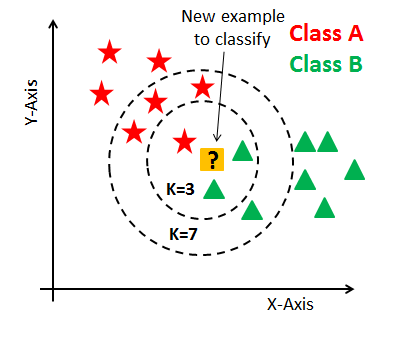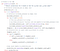Implementing k-Nearest Neighbors

Step by step instruction using k-NN (from scratch) in Python on the infamous Wine Quality Repository found on UCI: https://archive.ics.uci.edu/ml/datasets/Wine

So what is k-NN? According to Wikipedia, the k-NN algorithm is a non-parametric method used for classification and regression.

For classification, k-NN works by selecting specific examples closest to the query and grabs the most frequent label. So if k = 1, the object is assigned to the class of the single nearest neighbor. For regression, it averages the labels of the k nearest neighbors.Classification example. K=3 will choose triangle, while K=7 chooses the stars.

Now that we know what k-Nearest Neighbors is, let’s see how many steps it takes to compute…. 4! 4 easy steps.

1. Calculate Euclidean Distance
2. Get Nearest Neighbors (Fit)
3. Make Predictions
4. Display!

Step one involves finding the Euclidean distance. That is calculating the square root of the sum of the squared differences between two vectors. The smaller the value, the more similar the two records will be. If you receive a value of zero, there is no difference.

Next the input data is fit into X_train and y_train data. When you “fit” the data with k-NN, you are fitting a classifier by taking a dataset as input, then outputting a classifier, which is chosen from a space of possible classifiers. Basically, fitting k-NN requires storing the training set, and helps in optimization.

Then, we are going to make predictions by calculating distances between points, class predictions, and analyzing neighbors.The prediction section ties in the Euclidean Distance and Fit data

Lastly, a function for the display was created similar to the sklearn model. I will show an example of the Wine dataset using the sklearn model shortly.

Here is an example of k-NN on the Wine Dataset from UCI: